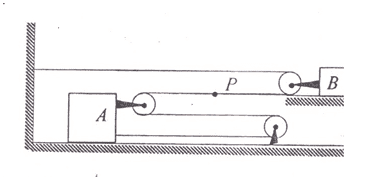# Multiple Pulleys problem

• Quantum Mind

## Homework Statement

The block B moves to the right with a constant velocity of 30 cm/s. Given this, which of the following is correct:

1. Speed of block A is 15 cm/s
2. Speed of point P is 60 cm/s
3. Speed of point P is 30 cm/s
4. Speed of block A is 40 cm/s

Fnet = ma ?

## The Attempt at a Solution

I don't know where to start. May be I will have to take each block separately, but I do not know the mass of either A or B nor the velocity of B.

http://www.pictureshack.us/images/346Q.png

## Homework Statement

The block B moves to the right with a constant velocity of 30 cm/s. Given this, which of the following is correct:

1. Speed of block A is 15 cm/s
2. Speed of point P is 60 cm/s
3. Speed of point P is 30 cm/s
4. Speed of block A is 40 cm/s

Fnet = ma ?

## The Attempt at a Solution

I don't know where to start. May be I will have to take each block separately, but I do not know the mass of either A or B nor the velocity of B.Put a few words in red to draw your attention to them.

The velocity of A is uniform and in the same direction, 30 cm/s to the right and therefore the acceleration is zero. Since acceleration is constant throughout the system, B should also move with uniform velocity (a = 0).

F = W = ma. Since a is zero, does this mean no work is done? How do I find the velocity of the block A or the point P?

The velocity of A is uniform and in the same direction, 30 cm/s to the right and therefore the acceleration is zero. Since acceleration is constant throughout the system, B should also move with uniform velocity (a = 0).

F = W = ma. Since a is zero, does this mean no work is done? How do I find the velocity of the block A or the point P?

This problem has nothing to do with Force, Work, Acceleration, mass or friction. It is all about speed -thus the distance traveled each unit of time.

In a short period of time, B will move 3 cm to the right [it is traveling at 30 cm/sec]
What happens to the length of the top string while that happens?
Where does that extra string come from or go to?
How far will P and A move in that same time then?

Given the options, you can hope that P moves either 6 or 3 cm, Or A moves 1.5 cm or 4 cm.
More importantly, only one of those had better happen or there is more than one correct answer.

I think the point P should also move with the same velocity as block B as the string at the top increases in length. I thought the answer would be choice (3), i.e. 30 cm/s, but apparently this is wrong and the answer is given as (2), i.e. 60 cm/s. This has me confused.

I think the point P should also move with the same velocity as block B as the string at the top increases in length. I thought the answer would be choice (3), i.e. 30 cm/s, but apparently this is wrong and the answer is given as (2), i.e. 60 cm/s. This has me confused.

If you put a reference point on the top string - call it Q - and consider the 3 cm move I suggested then ...

Q will be 3 cm further from the top pulley [Q is tied to the wall and the pulley is tied to B, which has moved 3 cm to the right].

That means the top string is 3 cm longer that it was.

That can only happen if 3 cm of string passes around the top pulley.

That will mean P moves 3 cm closer to the pulley; a pulley that has moved 3 cm.

Thus point P must have moved a total of 6 cm; meaning P is traveling at twice the speed of B - so 60cm/sec.

I get it now, thanks.